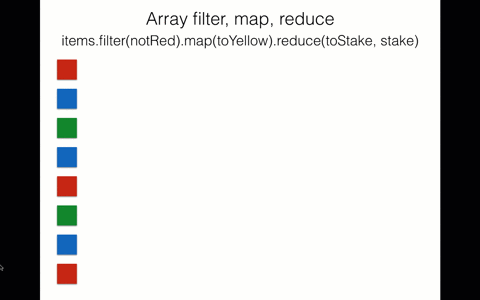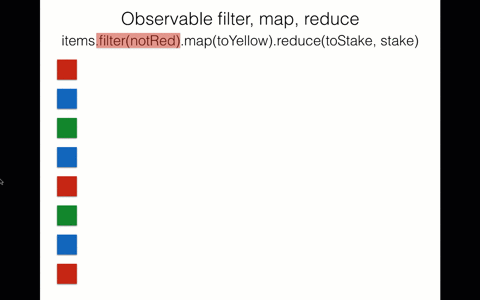#2017 iT 邦幫忙鐵人賽
DAY 22
3
Modern Web

# 30 天精通 RxJS(21): 深入 Observable

1. 延遲運算
2. 漸進式取值

## 延遲運算

``````var source = Rx.Observable.from([1,2,3,4,5]);
var example = source.map(x => x + 1);
``````

``````var source = [1,2,3,4,5];
var example = source.map(x => x + 1);
``````

## 漸進式取值

``````var source = [1,2,3];
var example = source
.filter(x => x % 2 === 0) // 這裡會運算並返回一個完整的陣列
.map(x => x + 1) // 這裡也會運算並返回一個完整的陣列
``````

``````Array.prototype.map = function(callback) {
var result = []; // 建立新陣列
this.forEach(function(item, index, array) {
result.push(callback(item, index, array))
});
return result; // 返回新陣列
}
``````Observable operator 的運算方式跟陣列的是完全的不同，雖然 Observable 的 operator 也都會回傳一個新的 observable，但因為元素是漸進式取得的關係，所以每次的運算是一個元素運算到底，而不是運算完全部的元素再返回。

``````var source = Rx.Observable.from([1,2,3]);
var example = source
.filter(x => x % 2 === 0)
.map(x => x + 1)

example.subscribe(console.log);
``````

1. 送出 `1` 到 filter 被過濾掉
2. 送出 `2` 到 filter 在被送到 map 轉成 `3`，送到 observer `console.log` 印出
3. 送出 `3` 到 filter 被過濾掉

``````class IteratorFromArray {
constructor(arr) {
this._array = arr;
this._cursor = 0;
}

next() {
return this._cursor < this._array.length ?
{ value: this._array[this._cursor++], done: false } :
{ done: true };
}

map(callback) {
const iterator = new IteratorFromArray(this._array);
return {
next: () => {
const { done, value } = iterator.next();
return {
done: done,
value: done ? undefined : callback(value)
}
}
}
}
}

var myIterator = new IteratorFromArray([1,2,3]);
var newIterator = myIterator.map(x => x + 1);
newIterator.next(); // { done: false, value: 2 }
``````## 今日小結

30 天精通 RxJS30

### 4 則留言

0

@小財神 我要怎麼插入 gif 的圖檔？

1

1

``````var myIterator = new IteratorFromArray([1,2,3]);
var newIterator = myIterator.map(x => x + 1);
newIterator.next(); // { done: false, value: 2 }
``````